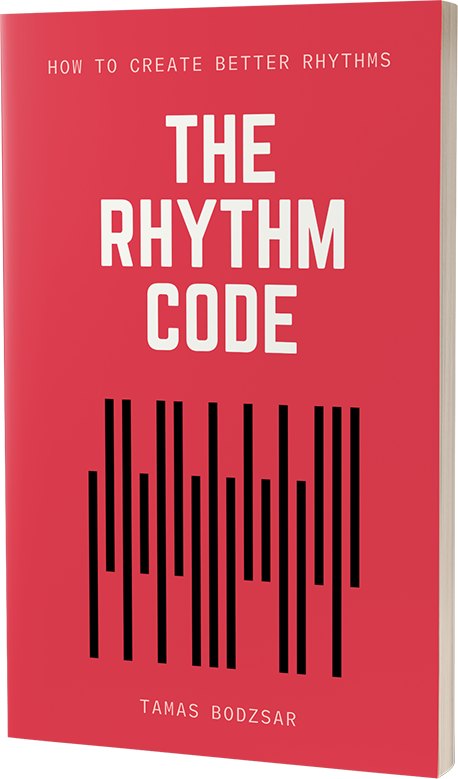Posted on

# Diatonic chordsIf you are a beginner songwriter, it’s the absolute best choice to use diatonic chords for writing a new song. This is because it’s easier to understand the relation between diatonic chords and create chord progressions with them. And generally, it’s easier to create a new song using only these chords.

So let’s take a look at what are diatonic chords.

We build diatonic chords when we use only the notes from within a key. A key is a system in tonality, and I like to call it a hidden system.

Why is it hidden? Because usually songs don’t use ALL the notes of the key (or at least not at the same time), yet all music listeners SENSE that there is a system. And if you know music theory, you know that we call this system “the key”. For example, we know that there are 7 notes in the key of C major, but we also know that we only play a few notes at a time.

So in the key of C major, we use these set of notes: C, D, E, F, G, A, B

We create diatonic chords using ONLY these notes! We can start creating these chords by using each of these notes as a root note. So first, if the root note of the chord is C, we add a third and a fifth to create the C major triad: C – E – G

The second note is the D, so if we add a third and a fifth (using only the notes of the scale!), we get a D minor chord: D – F – A

Here are all the diatonic chords if we create only triads:

C = C – E – G
Dm = D – F – A
Em = E – G – B
F = F – A – C
G = G – B – D
Am = A – C – E
Bdim = B – D – F

This is only an example in the key of C major! But since we have 12 keys, and each key contains 7 notes, there are 84 diatonic chords. However, if you choose a key for your song, you should stick to that key, and you will need to use only 7 diatonic chords.

## Diatonic Seventh Chords

Instead of triads, we can use seventh chords, so there are 7 diatonic seventh chords in a key. We can create a seventh chord by adding the seventh note to the triad. (The seventh note interval is always relative to the root note of the chord.)

Here are all the diatonic seventh chords in the key of C major:

Cmaj7 = C – E – G – B
Dm7 = D – F – A – C
Em7 = E – G – B – D
Fmaj7 = F – A – C – E
G7 = G – B – D – F
Am7 = A – C – E – G
Bm7b5 = B – D – F – A

## Diatonic Extended Chords

If we extend seventh chords, we can create extended chords: 9, 11, and 13 chords. Diatonic extended chords also contain only the notes of the key, however, some of the diatonic extended chords are useless because they sound bad!

Here are the diatonic extended chords, all the way up to 13 chords:

Cmaj13 = C – E – G – B – D – F – A
Dm13 = D – F – A – C – E – G – H
Em11(b9b13) = E – G – B – D – F – A – C
Fmaj13(#11) = F – A – C – E – G – B – D
G13 = G – B – D – F – A – C – E
Am11(b13) = A – C – E – G – B – D – F
Bm11(b5b9b13) = B – D – F – A – C – E – G

It might be confusing that these chord symbols contain “b” and “#” because these usually represent altered notes, and there are no altered notes in diatonic chords. These are not alterations, but this is the only way we can mark certain chord tones.

Let’s take a look at the chord Fmaj13(#11). If the chord symbol is Fmaj13, that would indicate a perfect 11 (or perfect fourth) chord tone, which is Bb. But since there is no Bb note in the key of C major, we need to indicate the chord tone B as #11.

So don’t confuse chord symbols with alterations, these are all diatonic chords.

Many of these chords don’t sound good, and this is because of the following reasons:

1. A perfect 11 chord tone doesn’t sound good in a major chord. So the Cmaj13 and the G13 diatonic chords are never used, instead, we always use the altered #11 if we use an extended major chord.

2. Minor chords don’t sound good with a flat 9 (b9), so that eliminates the Em11(b9b13), Am11(b13), and Bm11(b5b9b13) chords.

So the only useful diatonic extended chords are the Dm13 and the Fmaj13(#11), all the other chords can be used only with alterations.

Extended chords are mostly used in jazz, or if you want to make your chord progression sound jazzier. It’s tricky to use extended chords in songwriting, but I wrote a complete article about this: When to use extended chords in songwriting.

The secret pattern behind successful songs

Get the eBook for \$4.99